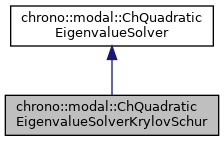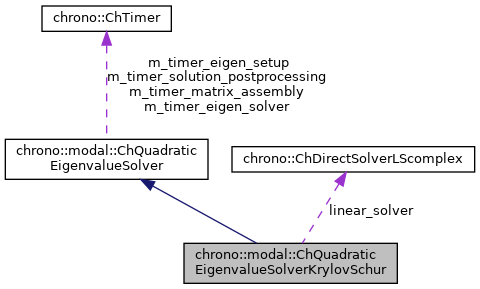## Description

Solves the eigenvalue problem with the Krylov-Schur iterative method.

This is an efficient method to compute only the lower n modes, ex. when there are so many degreees of freedom that it would make a full solution impossible. It uses an iterative method and it exploits the sparsity of the matrices.

#include <ChEigenvalueSolver.h>[legend][legend]

## Public Member Functions

Default: uses Eigen::SparseQR as factorization for the shift&invert, otherwise pass a custom complex sparse solver for faster factorization (ex. More...

virtual bool Solve (const ChSparseMatrix &M, const ChSparseMatrix &R, const ChSparseMatrix &K, const ChSparseMatrix &Cq, ChMatrixDynamic< std::complex< double >> &V, ChVectorDynamic< std::complex< double >> &eig, ChVectorDynamic< double > &freq, ChVectorDynamic< double > &damping_ratio, ChEigenvalueSolverSettings settings=0) const override
Solve the quadratic eigenvalue problem (lambda^2*M + lambda*R + K)*x = 0 s.t. More...Public Member Functions inherited from chrono::modal::ChQuadraticEigenvalueSolver
double GetTimeMatrixAssembly () const
Get cumulative time for matrix assembly.

double GetTimeEigenSetup () const
Get cumulative time eigensolver setup.

double GetTimeEigenSolver () const
Get cumulative time eigensolver solution.

double GetTimeSolutionPostProcessing () const
Get cumulative time for post-solver solution postprocessing.

## Public Attributes

ChDirectSolverLScomplexlinear_solverProtected Attributes inherited from chrono::modal::ChQuadraticEigenvalueSolver
ChTimer m_timer_matrix_assembly
timer for matrix assembly

ChTimer m_timer_eigen_setup
timer for eigensolver setup

ChTimer m_timer_eigen_solver
timer for eigensolver solution

ChTimer m_timer_solution_postprocessing
timer for conversion of eigensolver solution

## Constructor & Destructor Documentation

 chrono::modal::ChQuadraticEigenvalueSolverKrylovSchur::ChQuadraticEigenvalueSolverKrylovSchur ( ChDirectSolverLScomplex * mlinear_solver = 0 )

Default: uses Eigen::SparseQR as factorization for the shift&invert, otherwise pass a custom complex sparse solver for faster factorization (ex.

## ◆ Solve()

 bool chrono::modal::ChQuadraticEigenvalueSolverKrylovSchur::Solve ( const ChSparseMatrix & M, const ChSparseMatrix & R, const ChSparseMatrix & K, const ChSparseMatrix & Cq, ChMatrixDynamic< std::complex< double >> & V, ChVectorDynamic< std::complex< double >> & eig, ChVectorDynamic< double > & freq, ChVectorDynamic< double > & damping_ratio, ChEigenvalueSolverSettings settings = 0 ) const
overridevirtual

Solve the quadratic eigenvalue problem (lambda^2*M + lambda*R + K)*x = 0 s.t.

Cq*x = 0 Returns only the first lower n_modes. If n_modes=0, it return all eigenvalues (performance warning)

< output matrix with eigenvectors as columns, will be resized

< output vector with eigenvalues (real part not zero if some damping), will be resized

< 0 = k-th eigenvalue is real, 1= k-th and k-th+1 are complex conjugate pairs

< 0 = has converged, 1 = hasn't converged

< number of converged eigenvalues

< number of used iterations

< callback for A*v

< initial approx of eigenvector, or random

< size of A

< number of needed eigenvalues

< Krylov restart threshold (largest dimension of krylov subspace)

< max iteration number

< tolerance

Parameters
 M input M matrix R input R matrix K input K matrix Cq input Cq matrix of constraint jacobians V output matrix with eigenvectors as columns, will be resized eig output vector with complex eigenvalues (real part not zero if some damping), will be resized freq output vector with n frequencies [Hz], as f=w/(2*PI), will be resized. damping_ratio output vector with n damping rations r=damping/critical_damping. settings optional: settings for the solver, or n. of desired lower eigenvalues.